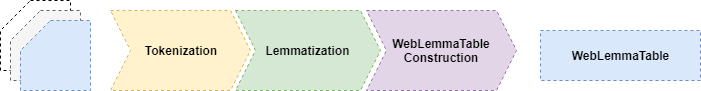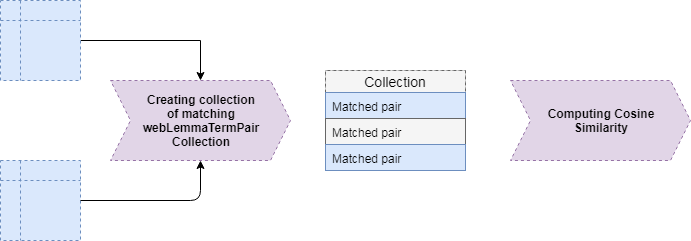# TF-IDF, SSRM and cSSRM document similarity

The page covers theoretical and practical aspects of document content similarity computation, using imbNLP Framework.
Let us first do theoretical overview on issue of document topical, or, semantic similarity.

VSM / TF-IDF topical modeling

The Vector Space Model (VSM) ⁠ defines documents and queries as vectors of identifiers in n-dimensional space, where each algebraic dimension corresponds to a distinct term, having non-zero weight attribute when it is part of queried document. The most common weight computation schema applied with VSM is the Term Frequency – Inverse Document Frequency (TF-IDF) ⁠

#### Traditional (the most popular) term weight computetion by TF-IDF

$$W_{ci} = \Big(\frac{F_i}{F_{max}} \cdot \frac{N}{n_i}\Big), ~ (1)$$

#### Cosine similarity computation within VSM

$$Sim(c,s) = \frac{ \sum_{n=1}^{n} Cw_i Sw_i }{\sqrt{ \sum_{n=1}^{n} {Cw_i}^2 \cdot \sum_{n=1}^{n} {Sw_i}^2 }} , C \in S, ~ (2)$$

In early stage of BEC research, we faced another critical issue of TF-IDF, relevant to all classification applications of the schema: the most common terms, shared among all documents of the training set for a class, will have weight set to 0 due to IDF. This counters the classification purpose, as co-occurrence of terms is expected feature among documents representing the same class.

A corrected TF-IDF: TF-cIDF

To overcome these issues, we introduced Document Frequency Correction (DFC) factor, by default set to 1.1. The factor reduces influence of IDF by multiplying number of documents (N) in equation (1):

$$W_{ci} = \Big(\frac{F_i}{F_{max}} \cdot \frac{N \cdot DFC}{n_i}\Big) (1)$$

where: Wci is term i weight in Lemma Table of category c, Fi is HTML-weighted frequency of the term i, Fmax is the highest HTML-weighted frequency in the set, N is number of documents, ni number of documents that include term i.

The TF-IDF based FVP uses term weighs from Lemma Table (1), directly, for both case and category terms. The similarity Sim(c,s), between category c and web site s, is defined as cosine function

$$Sim(c,s) = \frac{ \sum_{n=1}^{n} Cw_i Sw_i }{\sqrt{ \sum_{n=1}^{n} {Cw_i}^2 \cdot \sum_{n=1}^{n} {Sw_i}^2 }} , C \in S (2)$$

where: Cwi is category weight and Wsi is web site weight of the term i, n is number of common terms between the category Lemma Table and the web site Lemma Table. In respect to equation (1), both DFC=1 (plain TF-IDF) and DFC=1.1 are practically evaluated.

### Practical guide on TF-cIDF computationIn imbNLP.PartOfSpeech implementation (newer), Term Weights are computed during Term Table construction process, by table constructor. For WebLemmaTable it is: wlfConstructorTFIDF

In settings of the constructor, you’ll find properties to set value of the DFC, factors for HTML tags, if IDF should be even considered, and other stuff that should be set before using the constructor to Web Lemma Table construction.Steps for computing similarity between two Web Lemma Tables:

Returned value represents coefficient of similarity (double)

Example code:

// WLTableOfCategory and WLTableOfCase are webLemmaTermTable instance
var lemmaOverlap = WLTableOfIndustryCategory.GetMatchingTerms(WLTableOfCase);
Double similarity = lemmaOverlap.GetCosineSimilarity(logger);

Semantic Similarity Retrieval Model – SSRM

One of the general issues with the TF-IDF schema comes from term commonality requirement i.e. need for non empty set of mutual terms, between the document and query, in order to compute any similarity ratio other then zero. The issue is particularly relevant in context of languages with complex morphology, as the stemming process, supported with suitable morphosyntactic resources, becomes critical factor for successful implementation of the schema. One of the most popular approaches, addressing this issue, is the Semantic Similarity Retrieval Model (SSRM) ⁠ It is a semantically enhanced alternative to the VSM providing better approximation of human topical perception. In this model, after the query terms are expanded with synonyms, derived from a lexical ontology, their initial weights are calculated in typical TF-IDF fashion.

In the first step of SSRM, the query term weight qi ,of each query term i is adjusted according to its relationships with other semantically similar terms j within the same query vector:

$$q_i = q_i + \sum^{j \not= i}_{sim(i,j) \geq t} q_j \cdot sim(i,j) ~ (3)$$

where t is predefined threshold. Multiple related terms, in the same query, reinforce each other. The weights of non-similar terms remain unchanged. For short queries, specifying only a few terms, the weights are initialized to 1 and adjusted according to the formula above. In the second step, the query term is expanded up to three levels of ontological hierarchy, in both hyponym and hypernym direction. Weight for, newly added, terms is computed as:

$$q_i = \sum^{j \not= i}_{sim(i,j) \geq t} \frac{1}{n} \cdot q_j \cdot sim(i,j) ~ (4)$$

Document (d) vs query (q) similarity computation:

$$Sim(q,d) = \frac{ \sum_{i=1}^{i} \sum_{j=1}^{j} q_i d_j sim(i,j) } {\sum_{i=1}^{i} \sum_{j=1}^{j} q_i d_j} , q \in d (5)$$

Example code:

// Terms of the case
List<webLemmaTerm> caseTerms = caseKnowledge.WLTableOfIndustryClass.GetList();

// Creating expanded cloud
var expandedCloud = classKnowledge.semanticCloudFiltered.ExpandTermsToCloud(caseTerms, settings.caseTermExpansionSteps, true, settings.caseTermExpansionOptions);

// Makes overlap pairs
var lemmaOverlap = classKnowledge.semanticCloudFiltered.GetMatchingTerms(caseTerms, true);

// Computes similarity
Double similarity = expandedCloud.GetSSRM(lemmaOverlap, logger);

Cosine SSRM – cSSRM

I’ve proposed the cSSRM, a cosine similarity computation schema, based on SSRM ⁠ principles. It uses the similar term expansion and the same re-weighting procedure, with different similarity computation.

$$Sim(c,s) = \frac{ \sum_{n=1}^{n} Cw_i Sw_i sim(c_i, s_i) }{\sqrt{\sum_{n=1}^{n} {Sw_i}^2 \cdot\sum_{n=1}^{n} { ( Cw_i, sim(c_i, s_i) ) } ^2 }} , C \in S$$

Example code:

// Getting all case terms from WebLemmaTermTable, representing an Industry
List<webLemmaTerm> caseTerms = caseKnowledge.WLTableOfIndustryClass.GetList();

// Expands caseTerms using semantic cloud
List<webLemmaTerm> expandedTerms = classKnowledge.semanticCloudFiltered.ExpandTerms(caseTerms, settings.caseTermExpansionSteps, settings.caseTermExpansionOptions);

// Finds matched terms
var cloudOverlap = classKnowledge.semanticCloudFiltered.GetMatchingTerms(expandedTerms);

// This will return Cosine SSRM
Double similarity = cloudOverlap.GetCosineSimilarity(logger);

 G. Salton, A. Wong, C. Yang S., A vector space model for automatic indexing, Commun. ACM. 18 (1975) 613–620. doi:10.1145/361219.361220.

 K.S. Jones, A STATISTICAL INTERPRETATION OF TERM SPECIFICITY AND ITS APPLICATION IN RETRIEVAL, J. Doc. 28 (1972) 11–21. doi:10.1108/eb026526.

 A. Hliaoutakis, G. Varelas, E. Voutsakis, E.G.M. Petrakis, E. Milios, Information Retrieval by Semantic Similarity, Int. J. Semant. Web Inf. Syst. 2 (2006) 55–73. doi:10.4018/jswis.2006070104.

TF-cIDF and cSSRM computation schemata, proposed here, are part of an article currently under peer-review process. If you intend to cite these, please consider to wait a bit until the article is reviewed, so you have reference of higher credibility.